# 「时间」与「空间」复杂度

2018/10/18 15:07

• 时间维度：是指执行当前算法所消耗的时间，我们通常用「时间复杂度」来描述。

• 空间维度：是指执行当前算法需要占用多少内存空间，我们通常用「空间复杂度」来描述。

## 时间复杂度

### 大O符号表示法

• 相对(relative)：你只能比较相同的事物。你不能把一个做算数乘法的算法和排序整数列表的算法进行比较。但是，比较2个算法所做的算术操作（一个做乘法，一个做加法）将会告诉你一些有意义的东西；

• 表示(representation)：大O(用它最简单的形式)把算法间的比较简化为了一个单一变量。这个变量的选择基于观察或假设。例如，排序算法之间的对比通常是基于比较操作(比较2个结点来决定这2个结点的相对顺序)。这里面就假设了比较操作的计算开销很大。但是，如果比较操作的计算开销不大，而交换操作的计算开销很大，又会怎么样呢？这就改变了先前的比较方式；

• 复杂度(complexity)：如果排序10,000个元素花费了我1秒，那么排序1百万个元素会花多少时间？在这个例子里，复杂度就是相对其他东西的度量结果。

### 常见的时间复杂度量级

• 常数阶O(1)

• 线性阶O(n)

• 平方阶O(n²)

• 对数阶O(logn)

• 线性对数阶O(nlogn)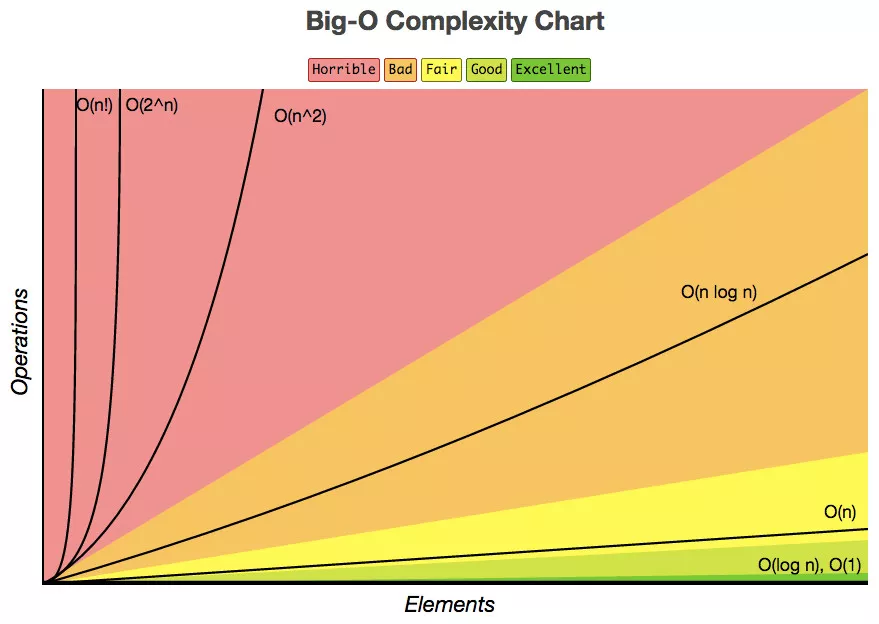#### O(1)void swapTwoInts(int &a, int &b){
int temp = a;
a = b;
b = temp;
}



#### O(n)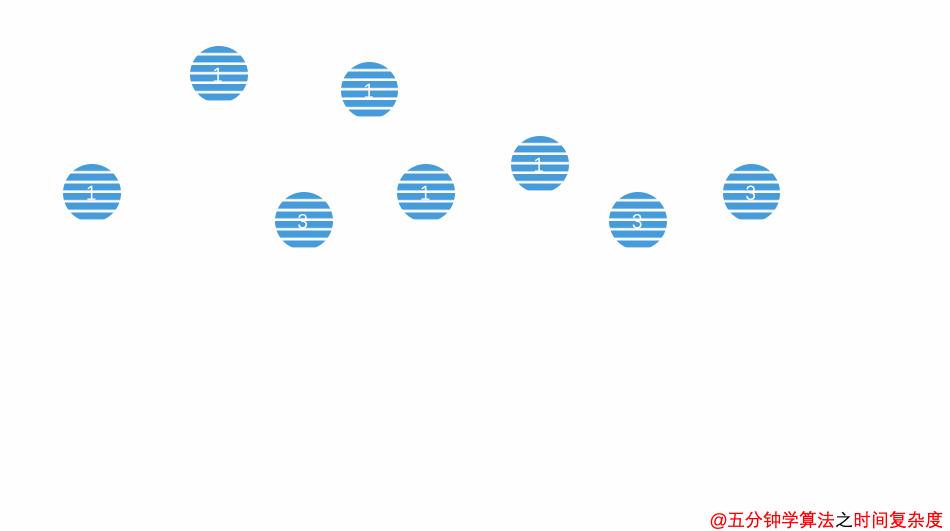int sum ( int n ){
int ret = 0;
for ( int i = 0 ; i <= n ; i ++){
ret += i;
}
return ret;
}



void reverse ( string &s ) {
int n = s.size();
for (int i = 0 ; i < n/2 ; i++){
swap ( s[i] , s[n-1-i]);
}
}



#### O(n²)void selectionSort(int arr[],int n){
for(int i = 0; i < n ; i++){
int minIndex = i;
for (int j = i + 1; j < n ; j++ )
if (arr[j] < arr[minIndex])
minIndex = j;

swap ( arr[i], arr[minIndex]);
}
}



• 当 i = 0 时，第二重循环需要运行 (n - 1)  次

• 当 i = 1 时，第二重循环需要运行 (n - 2)  次

• 。。。。。。

(n - 1) + (n - 2) + (n - 3) + ... + 0
= (0 + n - 1) * n / 2
= O (n ^2)



void printInformation (int n ){
for (int i = 1 ; i <= n ; i++)
for (int j = 1 ; j <= 30 ; j ++)
cout<< "Hello,五分钟学算法：）"<< endl;
}



#### O(logn)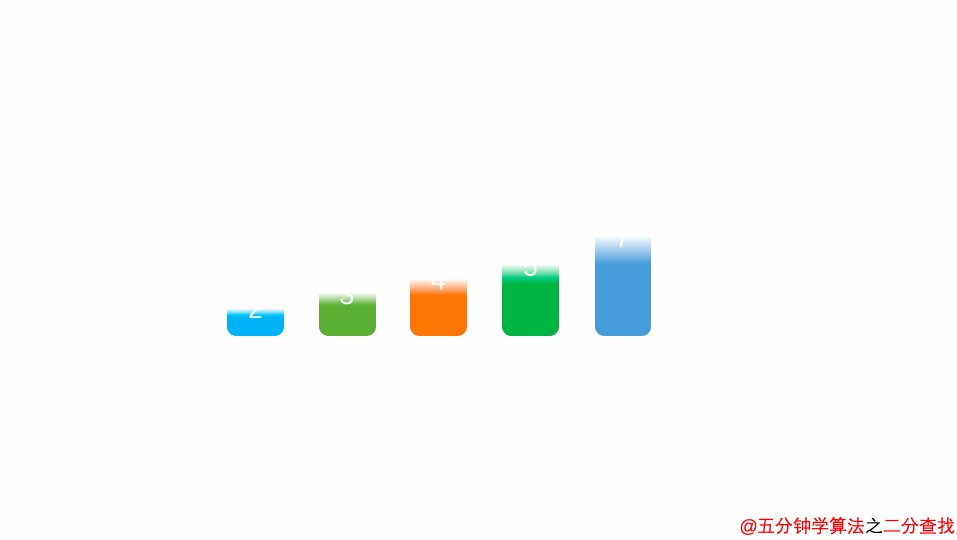int binarySearch( int arr[], int n , int target){
int l = 0, r = n - 1;
while ( l <= r) {
int mid = l + (r - l) / 2;
if (arr[mid] == target) return mid;
if (arr[mid] > target ) r = mid - 1;
else l = mid + 1;
}
return -1;
}



// 整形转成字符串
string intToString ( int num ){
string s = "";
// n 经过几次“除以10”的操作后，等于0
while (num ){
s += '0' + num%10;
num /= 10;
}
reverse(s)
return s;
}
void hello (int n ) {
// n 除以几次 2 到 1
for ( int sz = 1; sz < n ; sz += sz)
for (int i = 1; i < n; i++)
cout<< "Hello,五分钟学算法：）"<< endl;
}

#### O(nlogn)

void hello (){
for( m = 1 ; m < n ; m++){
i = 1;
while( i < n ){
i = i * 2;
}
}
}

### 不常见的时间复杂度

#### 递归算法的时间复杂度

① 递归中进行一次递归调用的复杂度分析int binarySearch(int arr[], int l, int r, int target){
if( l > r ) return -1;

int mid = l + (r-l)/2;
if( arr[mid] == target ) return mid;
else if( arr[mid] > target )
return binarySearch(arr, l, mid-1, target);    // 左边
else
return binarySearch(arr, mid+1, r, target);   // 右边
}int sum (int n) {
if (n == 0) return 0;
return n + sum( n - 1 )
}//递归深度：logn
//时间复杂度：O(logn)
double pow( double x, int n){
if (n == 0) return 1.0;

double t = pow(x,n/2);
if (n %2) return x*t*t;
return t * t;
}

② 递归中进行多次递归调用的复杂度分析

// O(2^n) 指数级别的数量级，后续动态规划的优化点
int f(int n){
if (n == 0) return 1;
return f(n-1) + f(n - 1);
}1 + 2 + 4 + 8 = 15

2^0 + 2^1 + 2^2 + …… + 2^n
= 2^(n+1) - 1
= O(2^n)• 1. 上述例子中树的深度为n，而 归并排序 的递归树深度为logn

• 2. 上述例子中每次处理的数据规模是一样的，而在 归并排序 中每个节点处理的数据规模是逐渐缩小的

#### 最好、最坏情况时间复杂度int find(int[] array, int n, int x) {
for (  int i = 0 ; i < n; i++) {
if (array[i] == x) {
return i;
break;
}
}
return -1;
}

#### 平均情况时间复杂度

((1 + 2 + … + n) / n + n)  /  2 = (3n + 1) / 4

find函数的平均时间复杂度为 O(n)。

#### 均摊复杂度分析template <typename T>
class MyVector{
private:
T* data;
int size;       // 存储数组中的元素个数
int capacity;   // 存储数组中可以容纳的最大的元素个数
// 复杂度为 O(n)
void resize(int newCapacity){
T *newData = new T[newCapacity];
for( int i = 0 ; i < size ; i ++ ){
newData[i] = data[i];
}
data = newData;
capacity = newCapacity;
}
public:
MyVector(){
data = new T;
size = 0;
capacity = 100;
}
// 平均复杂度为 O(1)
void push_back(T e){
if(size == capacity)
resize(2 * capacity);
data[size++] = e;
}
// 平均复杂度为 O(1)
T pop_back(){
size --;
return data[size];
}

};

push_back实现的功能是往数组的末尾增加一个元素，如果数组没有满，直接往后面插入元素；如果数组满了，即size == capacity，则将数组扩容一倍，然后再插入元素。

## 空间复杂度

(1) 固定部分，这部分空间的大小与输入/输出的数据的个数多少、数值无关。主要包括指令空间（即代码空间）、数据空间（常量、简单变量）等所占的空间。这部分属于静态空间。

(2) 可变空间，这部分空间的主要包括动态分配的空间，以及递归栈所需的空间等。这部分的空间大小与算法有关。

### 平衡二叉树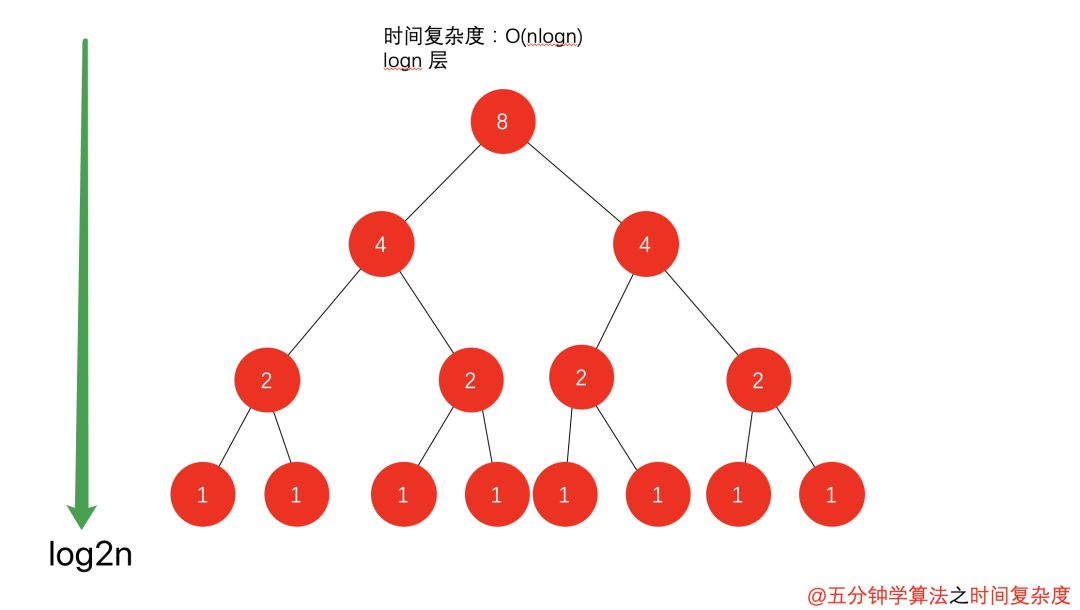### 列表二叉树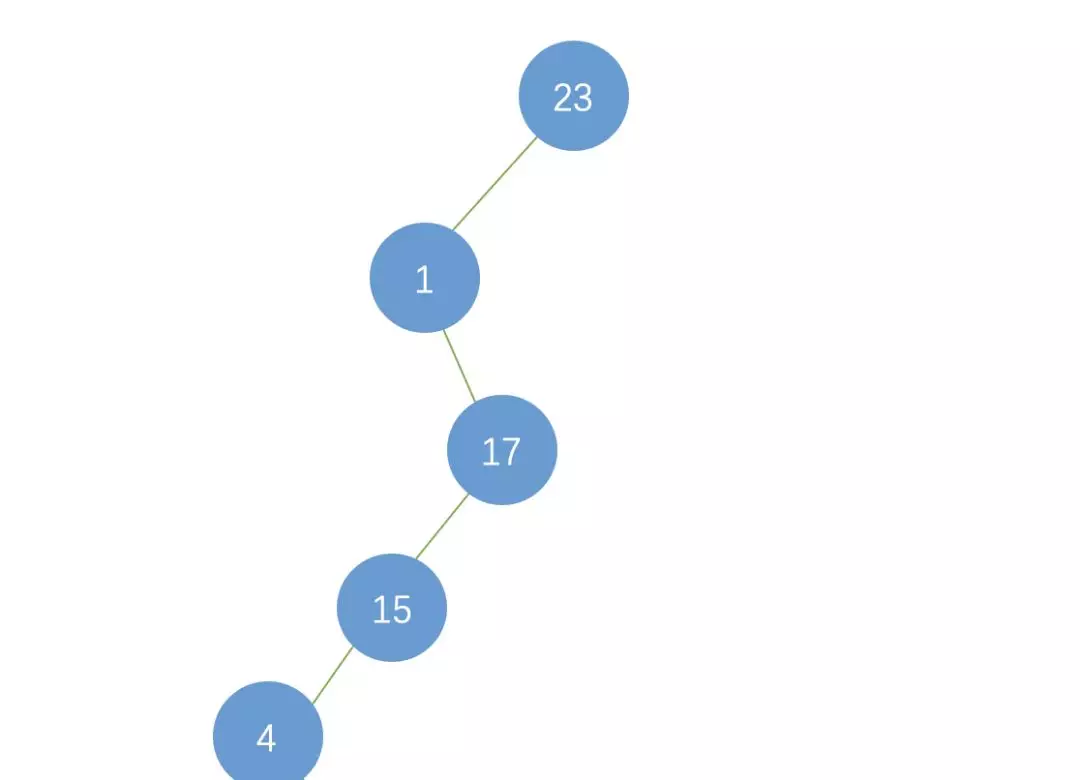## 冰火交融

• 1. 可以编写一个算法来计算，这也就意味着，每次给一个年份，都是要通过计算得到是否是闰年的结果。

• 2. 还有另一个办法就是，事先建立一个有 5555 个元素的数组（年数比现实多就行），然后把所有的年份按下标的数字对应，如果是闰年，此数组项的值就是1，如果不是值为0。这样，所谓的判断某一年是否是闰年，就变成了查找这个数组的某一项的值是多少的问题。此时，我们的运算是最小化了，但是硬盘上或者内存中需要存储这 5555 个 0 和 1 。

0
0 收藏

### 作者的其它热门文章0 评论
0 收藏
0